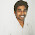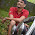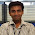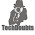# JWS Implementation in Asp.Net c#

JSON Web Signature is a commonly used web security method for encryption and decryption. Ww will see here, how to validate JWS using RSA public key encryption in ASP.NET.

## What's RSA Algorithm?

RSA algorithm is a commonly used encryption method to send or receive information securely. The above picture depicts RSA public key encryption. Original information can be encrypted with a private key, also that could be decrypted with public key (both these keys are different.).

## Overall Process

Here we receive a long encrypted text called token. This token contains three parts called header, payload and signature. Header provides which algorithm is used for encryption and its type. Payload contains original information like name, email id, mobile number etc. Signature is the private key encrypted form of header+payload. Which means

Now here we need to encrypt the header and payload using given public key, after that we need to check both the results are same. If the results are same, validation is successful otherwise its not successful. That is here we need to check following

## How to validate in asp.net c#?

First we need a Base64Url Decode method to get the correct form of given data. Because the idtoken consists of some extra characters like hyphen (-), equal to (=) etc. So first include below code in your program.

```   /// <summary>
/// Modify encrypted text to Base64 pattern and convert to bytes.
/// </summary>
/// <param name="input">Encrypted data as string.</param>
/// <returns>Base64 string as byte array.</returns>
private static byte[] Base64UrlDecode(string input)
{
var output = input;
output = output.Replace('-', '+'); // 62nd char of encoding
output = output.Replace('_', '/'); // 63rd char of encoding
switch (output.Length % 4) // Pad with trailing '='s
{
case 0: break; // No pad chars in this case
case 2: output += "=="; break; // Two pad chars
case 3: output += "="; break; // One pad char
default: throw new System.Exception("Illegal base64url string!");
}
var converted = Convert.FromBase64String(output); // Standard base64 decoder
return converted;
}
```

RSA public key consists of exponent and modulus and that will be given from your encryption manager. By using token information , public key exponent and modulus we could verify the information. Just include following two methods.

``` private bool VerifyTokenDetails(string idToken,string exponent,string modulus)
{
try
{
var parts = idToken.Split('.');
var header = parts;
var payload = parts;
string signedSignature = parts;
//Extract user info from payload
string userInfo = Encoding.UTF8.GetString(Base64UrlDecode(payload));
byte[] keyBytes = Base64UrlDecode(modulus);
string keyBase = Convert.ToBase64String(keyBytes);
string key = @"<RSAKeyValue> <Modulus>" + keyBase + "</Modulus> <Exponent>" + exponent + "</Exponent> </RSAKeyValue>";
bool result = VerifyData(originalMessage, signedSignature, key);
if (result)
return true;
else
return false;
}
catch (Exception ex) { }
return false;
}
/// <summary>
/// Verifies encrypted signed message with public key encrypted original message.
/// </summary>
/// <param name="originalMessage">Original message as string. (Encrypted form)</param>
/// <param name="signedMessage">Signed message as string. (Encrypted form)</param>
/// <param name="publicKey">Public key as XML string.</param>
/// <returns>Boolean True if successful otherwise return false.</returns>
private static bool VerifyData(string originalMessage, string signedMessage, string publicKey)
{
bool success = false;
using (var rsa = new RSACryptoServiceProvider())
{
var encoder = new UTF8Encoding();
byte[] bytesToVerify = encoder.GetBytes(originalMessage);
byte[] signedBytes = Base64UrlDecode(signedMessage);
try
{
rsa.FromXmlString(publicKey);
SHA256Managed Hash = new SHA256Managed();
byte[] hashedData = Hash.ComputeHash(signedBytes);
success = rsa.VerifyData(bytesToVerify, CryptoConfig.MapNameToOID("SHA256"), signedBytes);
}
catch (CryptographicException e)
{
success = false;
}
finally
{
rsa.PersistKeyInCsp = false;
}
}
return success;
}
```

Method VerifyData will return boolean true if the validation is success otherwise it will return false. Also here used SHA256 hash, but you can use your own hash method here. If you have any doubts or suggestions please comment here, let me know it.
JSON Web Signature (JWS) RSA Public Key Validation Asp.Net C#Reviewed by Biby on 8:44 AM Rating: 5

1.Thanks a Lot.. but why there is a need for Hash.ComputeHash(signedBytes); seems ur not using it anywhere

1.That is because of, to explain how to get hash data if there is needed. Some people need hashed data. Normally we don't need to use it. Did you tried this? is it worked?

2.1.you mean expiration time?

2.you can validate it using the "exp" from the payload. The exp is in epoch so you have to do some conversion before that.

3.have u done that?

4.if (IsEpochTimeValid(Convert.ToInt64(tokenPayload.nbf), Convert.ToInt64(tokenPayload.exp)) == false) return false;

------
private bool IsEpochTimeValid(long epochStart, long epochEnd)
{
long epochNow;

epochNow = ToUnixTime(DateTime.UtcNow);
if (epochNow <= epochStart || epochNow >= epochEnd)
{
return false;
}

return true;
}

5.Basically ignore my conversion of tokenPayload.nbf and tokenPayload.exp since its my own class. the important part is u convert the current time to epoch by following below conversion,

DateTime epochDate= new DateTime(1970, 1, 1, 0, 0, 0, DateTimeKind.Utc);
long epochNow = Convert.ToInt64((DateTime.UtcNow - epoch).TotalSeconds);

then voila, you can do the comparison.

6.Informative. Thank you @bizzare... :)

3.This article really helpful. Been searching high and low on how to validate jwt with rsa public key.

1.And I think you got solution... right? :)

2.4.Hi, I have been trying to verify my JWT but above provided solution isnt working for me.